# Texas Go Math Grade 3 Lesson 10.1 Answer Key Model Division

Refer to our Texas Go Math Grade 3 Answer Key Pdf to score good marks in the exams. Test yourself by practicing the problems from Texas Go Math Grade 3 Lesson 10.1 Answer Key Model Division.

## Texas Go Math Grade 3 Lesson 10.1 Answer Key Model Division

Essential Question
How can you use the strategy act it out to solve problems with equal groups?
To use the strategy act it out to solve problems with equal groups.
Materials, Math Board, counters etc.,

Unlock the Problem
Stacy has 16 flowers. She puts an equal number of flowers in each of 4 vases. How many flowers does Stacy put in each vase?
Use the graphic organizer below to solve the problem.
Stacy puts 4 flowers in each vase.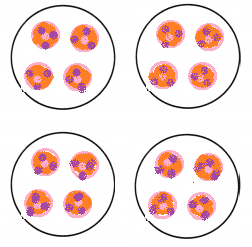Explanation:
Stacy has 16 flowers.
She puts an equal number of flowers in each of 4 vases.
Total flowers Stacy put in each vase 16 ÷ 4 = 4

What do I need to find?
I need to find the number of ___ Stacy puts in each ____.
I need to find the number of flowers Stacy puts in each vase.

What information am I given?
Stacy has 16 flowers.
She puts an equal number of flowers in each of 4 vases.

Plan
What is my plan or strategy?
I will act out the problem by making equal number of counters.

Solve

Describe how to act out the problem to solve.

First, count out 4 counters.
Next, make 4 equal groups. Place 1 counter at a time in each group until all 16 counters are used.

Last, draw the equal groups by completing the picture below.So, Stacy puts ___ flowers in each vase.So, Stacy puts 4 flowers in each vase

Try Another Problem

Hayden is planning a party. He bakes 21 cookies. If he plans to give each person 3 cookies, how many people will be at his party?
7 people
21 ÷ 3 = 7
Explanation:
If he plans to give each person 3 cookies,
Total people at his party
21 ÷ 3 = 7

What do I need to find?
I need to find number of people in the party.

What information am I given?
21 ÷ 3 = 7 persons

Plan
What is my plan or strategy?
To use the strategy act it out to solve problems with equal groups.
Explanation:
Dividing the 21 cookies with 3 cookies per person = 7 persons

Solve
Describe how to act out the problem to solve.
A problem-solving strategy is a plan of action used to find a solution.
Explanation:
Different strategies have different action plans associated with them to solve.

When solving a math problem, we can check if the answer we have derived to is reasonable or not, based on an estimate.
Explanation:
Rounding numbers, making numbers compatible, properties of operations are some strategies that are used to chec k the reasonableness of answers.

Math Talk
Mathematical Processes
Explain how acting out of a problem helps you solve it.
It helps to identify the problem to solve.
Explanation:
For example,
Underlying important facts.
Choose correct strategy.
Trial and Error method.
Using math board.
Unlock the Problem

✓ Use the Problem Solving Math Board.
✓ Underline important facts.
✓ Choose a strategy you know.

Share and Show

Question 1.
Sue is having a party. She has 16 cups. She puts them in 2 equal stacks. How many cups are in each stack?
First, decide how to act out the problem.
You can use counters to represent the ___.
You can draw ___ to represent the stacks.
Then, draw to find the number of ___ in each stack.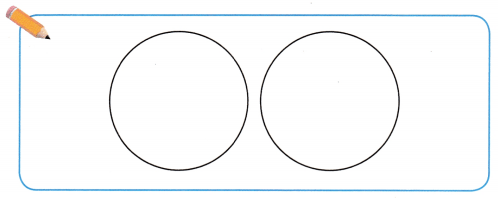There are ___ groups. There are ___ counters in each group.
So, there are ___ cups in each stack.
You can use counters to represent the CUPs.
You can draw Circles to represent the stacks.
Then, draw to find the number of cups in each stack.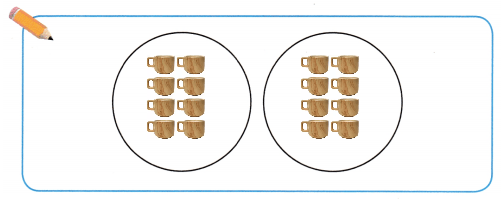There are 2 groups. There are 8 counters in each group.
So, there are 8 cups in each stack.

Quentin 2.
What if Sue has 24 cups and puts 4 cups in each stack? If she already made 4 stacks, how many more stacks can she make with the remaining cups?
2 more stacks with remaining cups.
Explanation:
Sue has 24 cups and puts 4 cups in each stack.
24 ÷ 4 = 6 stacks
2 more stacks she has to make with the remaining cups

Problem Solving

Question 3.
H.O.T. Multi-Step At Luke’s school party, the children get into teams of 5 to play a game. If there are 20 boys and 15 girls, how many teams are there?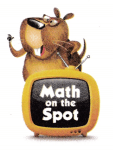7 teams.
Explanation:
The children get into teams of 5 to play a game.
If there are 20 boys and 15 girls.
Total boys and girls = 20 + 15 = 35
Total number of teams
35 ÷ 5 = 7

Question 4.
H.O.T. Evaluate You have 12 cookies. How many ways can the cookies be put equally on any number of plates?
12 ÷ 2 = 6
12 ÷ 3 = 4
12 ÷ 4 = 3
12 ÷ 6 = 2
Explanation:
Cookies can be placed equally in different number of plates as shown above.

Fill in the bubble for the correct answer choice.
You may use objects or models to solve.

Question 5.
Angie is having a tie-dye party. There are 20 T-shirts in 4 buckets of dye. There is the same number of T-shirts in each bucket. How many T-shirts are in each bucket?
(A) 16
(B) 8
(C) 5
(D) 24
Explanation:
There are 20 T-shirts in 4 buckets of dye.
Number of T-shirts in each bucket
20 ÷ 4 = 5

Question 6.
Analyze Gabriel has 27 fish in 3 tanks. He has the same number of fish in each tank. How many fish are in each tank?
(A) 9
(B) 24
(C) 30
(D) 8
Option (A)
Explanation:
Gabriel has 27 fish in 3 tanks.
He has the same number of fish in each tank.
Number of fish in each tank
27 ÷ 3 = 9

Question 7.
Multi-Step Ira and his brother share a model car collection. Ira has 25 cars, and his brother has 15 cars. They store the model cars on a bookshelf, and place the same number of cars on each shelf. There are 5 shelves. How many model cars are on each shelf?
(A) 35
(B) 40
(C) 5
(D) 8
Option (C)
Explanation:
Ira has 25 cars, and his brother has 15 cars.
There are 5 shelves.
Number of model cars are on each shelf
25 ÷ 5 = 5

Texas Test Prep

Question 8.
Apply Miguel bought 18 party favors. He gave 2 party favors to each of the children at his party. How many
children were at Miguel’s party?
(A) 8
(B) 16
(C) 9
(D) 20
Option (C)
Explanation:
Miguel bought 18 party favors.
He gave 2 party favors to each of the children at his party.
Number of children at Miguel’s party
18 ÷ 2 = 9

### Texas Go Math Grade 3 Lesson 10.1 Homework and Practice Answer Key

Draw to solve the problem.

Question 1.
Greg has 12 marbles. He puts them in 3 equal groups. How many marbles are in each group?
There are __ marbles.
There are ___ groups.
So, there are ___ in each group.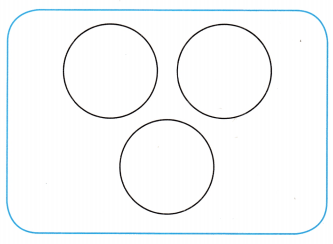There are 12 marbles.
There are 3 groups.
So, there are 4 in each group.
Explanation:
Greg has 12 marbles.
He puts them in 3 equal groups.
Number of marbles in each group 12 ÷ 3 = 4

Question 2.
Yuri has 12 counters. He puts them in 2 equal groups. How many counters are in each group?
There are __ counters.
There are ___ groups.
So, there are ___ in each group.There are 12 counters.
There are  2 groups.
So, there are 6 in each group.
Explanation:
Yuri has 12 counters.
He puts them in 2 equal groups.
12 ÷ 6 =2
Each group is equally distributed with 6 counters.Explanation:
Total 12 counters are equally distributed in 2 groups.

Problem Solving

Question 3.
A farmer puts an equal number of fruit in each of 6 baskets. If there are 18 pears and 24 peaches, how many pieces of fruit are in each basket?
7 pieces of fruits in each basket.
Explanation:
A farmer puts an equal number of fruit in each of 6 baskets.
If there are 18 pears , it is distributed as 18 ÷ 6 = 3
and 24 peaches, is distributed as 24 ÷ 6 = 4
Number of pieces of fruits in each basket
3 + 4 = 7

Question 4.
The same farmer has 16 pumpkins. How many different ways can the pumpkins be put into equal groups?
16 ÷  4 = 4
16 ÷  8 = 2
16 ÷  2 = 8
Explanation:
The farmer has 16 pumpkins.
Different ways can the pumpkins be put into equal groups
16 ÷  4 = 4
16 ÷  8 = 2
16 ÷  2 = 8

Texas Test Prep

Lesson Check

Question 5.
Gordy makes 24 muffins. Each tray has 6 muffins. How many trays does Gordy have?
(A) 18
(B) 6
(C) 4
(D) 8
Option (C)
Explanation:
Gordy makes 24 muffins.
Each tray has 6 muffins.
Total trays Gordy have
24 ÷ 6 = 4

Question 6.
Sonja has 28 photos in an album. Each page has 4 photos. How many pages have photos?
(A) 9
(B) 24
(C) 8
(D) 7
Option (D)
Explanation:
Sonja has 28 photos in an album.
Each page has 4 photos.
Total pages of photos
28 ÷  4 = 7

Question 7.
Hunan works at the school breakfast party. He makes 30 pancakes. If he puts 5 pancakes on each plate, how many plates will he need?
(A) 4
(B) 6
(C) 8
(D) 25
Option (B)
Explanation:
Hunan makes 30 pancakes.
If he puts 5 pancakes on each plate,
Number of plates she need
30 ÷ 5 = 6

Question 8.
Cara works in a cycle shop. She has 18 wheels for tricycles. If each tricycle needs 3 wheels, how many tricycles can she put together?
(A) 21
(B) 5
(C) 15
(D) 6
Option (D)
Explanation:
Cara has 18 wheels for tricycles.
If each tricycle needs 3 wheels,
number of tricycles she put together
18 ÷ 3 = 6

Question 9.
Multi-Step Jason has 15 redheads and 10 blue beads. He makes 5 bracelets with an equal number of beads. How many beads are on each bracelet?
(A) 3
(B) 2
(C) 5
(D) 4
Option (C)
Explanation:
Total beads = 15 + 10 = 25
He makes 5 bracelets with an equal number of beads.
25 ÷ 5 = 5
Total beads on each bracelet are 5

Question 10.
Multi-Step Shana has 23 comic books and 19 coloring books. She puts them into 6 equal stacks. How many books are in each stack?
(A) 7
(B) 8
(C) 42
(D) 6